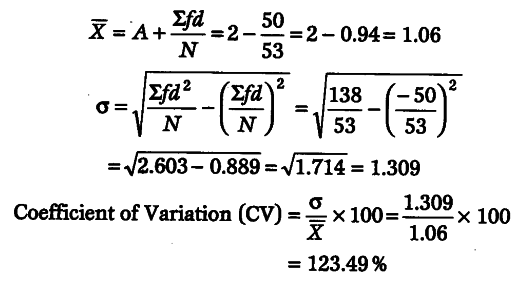# Calculate coefficient of variation from the following data

Calculate coefficient of variation from the following data

Calculation of Coefficient of Variation